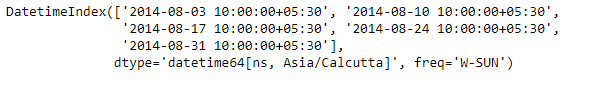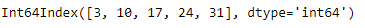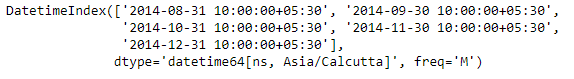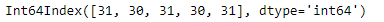Skip to content
Related Articles
Python | Pandas DatetimeIndex.day
• Last Updated : 24 Dec, 2018

Python is a great language for doing data analysis, primarily because of the fantastic ecosystem of data-centric python packages. Pandas is one of those packages and makes importing and analyzing data much easier.

Pandas` DatetimeIndex.day` attribute outputs an Index object containing the days in each of the entries of the DatetimeIndex object.

Syntax: DatetimeIndex.day

Return: Index containing days.

Example #1: Use `DatetimeIndex.day` attribute to find the days present in the DatetimeIndex object.

 `# importing pandas as pd``import` `pandas as pd`` ` `# Create the DatetimeIndex``# Here the 'W' represents Weekly frequency``didx ``=` `pd.DatetimeIndex(start ``=``'2014-08-01 10:00'``, freq ``=``'W'``,``                            ``periods ``=` `5``, tz ``=``'Asia/Calcutta'``)`` ` `# Print the DatetimeIndex``print``(didx)`

Output :Now we want to find all the day values present in the DatetimeIndex object.

 `# find all the days present in the object``didx.day`

Output :As we can see in the output, the function has returned an Index object containing the days value corresponding to days of each entry in the DatetimeIndex object.

Example #2: Use `DatetimeIndex.day` attribute to find the days present in the DatetimeIndex object.

 `# importing pandas as pd``import` `pandas as pd`` ` `# Create the DatetimeIndex``# Here the 'M' represents Month end frequency``didx ``=` `pd.DatetimeIndex(start ``=``'2014-08-01 10:00'``, freq ``=``'M'``, ``                          ``periods ``=` `5``, tz ``=``'Asia/Calcutta'``)`` ` `# Print the DatetimeIndex``print``(didx)`

Output :Now we want to find all the day values present in the DatetimeIndex object.

 `# find all the months in the object``didx.day`

Output :As we can see in the output, the function has returned an Index object containing the days value corresponding to days of each entry in the DatetimeIndex object.

Attention geek! Strengthen your foundations with the Python Programming Foundation Course and learn the basics.

To begin with, your interview preparations Enhance your Data Structures concepts with the Python DS Course. And to begin with your Machine Learning Journey, join the Machine Learning – Basic Level Course

My Personal Notes arrow_drop_up